# PSEB 6th Class Maths Solutions Chapter 5 Fractions Ex 5.3

Punjab State Board PSEB 6th Class Maths Book Solutions Chapter 5 Fractions Ex 5.3 Textbook Exercise Questions and Answers.

## PSEB Solutions for Class 6 Maths Chapter 5 Fractions Ex 5.3

1. Write the fraction for the shaded part and check whether these fractions are equivalent or not?

Question (i)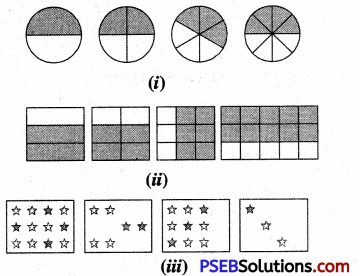Solution: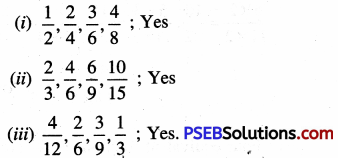2. Find four equivalent fractions of the followings:

Question (i)
(i) $$\frac {1}{4}$$
(ii) $$\frac {3}{5}$$
(iii) $$\frac {7}{9}$$
(iv) $$\frac {5}{11}$$
(v) $$\frac {2}{3}$$
Solution: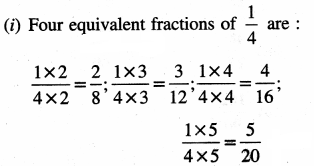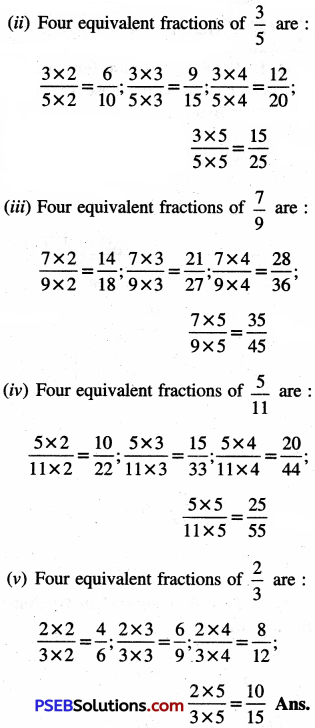3. Write the lowest equivalent fraction (simplest form) of :

Question (i)
(i) $$\frac {10}{25}$$
(ii) $$\frac {27}{54}$$
(iii) $$\frac {48}{72}$$
(iv) $$\frac {150}{60}$$
(v) $$\frac {162}{90}$$
Solution: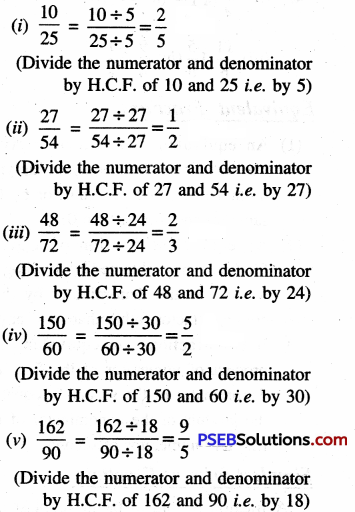4. Are the following fractions equivalent or not?

Question (i)
$$\frac{5}{12}, \frac{25}{60}$$
Solution:
We have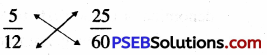By cross product,
5 × 60 = 300 and 12 × 25 = 300
Since two cross products are same
So, the given fractions are equivalent.Question (ii)
$$\frac{6}{7}, \frac{36}{42}$$
Solution:
We have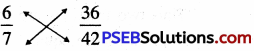By cross product,
6 × 42 = 252 and 7 × 36 = 252
Since two cross products are same
So, the given fractions are equivalent.

Question (iii)
$$\frac{7}{9}, \frac{56}{72}$$
Solution:
We have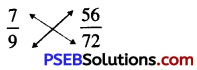By cross product,
7 × 72 = 504 and 9 × 56 = 504
Since two cross products are same
So, the given fractions are equivalent.

5. Replace [ ] 1 in each of the following by the correct number.

Question (i)
$$\frac{2}{7}$$ = 12 / [ ]
Solution:
Observe the numerators we have 12 ÷ 2 = 6
So, we multiply both numerator and denominator of $$\frac {2}{7}$$ by 6
We get $$\frac{2}{7}=\frac{2 \times 6}{7 \times 6}=\frac{12}{42}$$
Hence, the correct number in [ ] 1 is 42

Question (ii)
$$\frac{5}{8}$$ = 35 / [ ]
Solution:
Observe the numerators we have 35 ÷ 5 = 7
So, we multiply both numerator and denominator of $$\frac {5}{8}$$ by 7
We get $$\frac{5}{8}=\frac{5 \times 7}{8 \times 7}=\frac{35}{56}$$
Hence, the correct number in [ ] is 56.

Question (iii)
$$\frac{24}{36}$$ = 6 / [ ]
Solution:
Observe the numerators we have 24 ÷ 6 = 4
So, we divide both numerator and denominator of $$\frac {24}{36}$$ by 4
We get $$\frac{24}{36}=\frac{24 \div 4}{36 \div 4}=\frac{6}{9}$$
Hence, the correct number in [ ] is 9Question (iv)
$$\frac{30}{48}$$ = 8 / [ ]
Solution:
Observe the denominators we have 48 ÷ 8 = 6
So, we divide both numerator and denominator of $$\frac {30}{48}$$ by 6
We get $$\frac{30}{48}=\frac{30 \div 6}{48 \div 6}=\frac{5}{8}$$
Hence, the correct number in ⊇ is 5

Question (v)
$$\frac{7}{4}$$ = 42 / [ ]
Solution:
Observe the numerators we have 42 ÷ 7 = 6
So, we multiply both numerator and denominator of $$\frac {7}{4}$$ by 6
We get $$\frac{7}{4}=\frac{7 \times 6}{7 \times 6}=\frac{42}{24}$$
Hence, the correct number in [ ] is 24

6. Find the equivalent fraction of $$\frac {3}{5}$$, having

Question (i)
numerator 18
Solution:
(i) Equivalent fraction of $$\frac {3}{5}$$, having numerator 18 is
$$\frac{3}{5}$$ = 18 / [ ]
Observe the numerators, we have 18 ÷ 3=6
So, we multiply both numerator and denominator of $$\frac {3}{5}$$ by 6
∴ $$\frac{3}{5}=\frac{3 \times 6}{5 \times 6}=\frac{18}{30}$$
Thus, required equivalent fraction of $$\frac{3}{5}=\frac{18}{30}$$Question (ii)
denominator 20
Solution:
Equivalent fraction of $$\frac {3}{5}$$, having denominator 20 is $$\frac{3}{5}$$ = [ ] / 20
Observe the denominators, we have 20 ÷ 5 = 4
So, we multiply both numerator and denominator of $$\frac {3}{5}$$ by 4
Thus, required equivalent fraction of $$\frac{3}{5}=\frac{12}{20}$$
∴ $$\frac{3}{5}=\frac{3 \times 4}{5 \times 4}=\frac{12}{20}$$
Thus, required equivalent fraction of
$$\frac{3}{5}=\frac{12}{20}$$

Question (iii)
numerator 24.
Solution:
Equivalent fraction of $$\frac {3}{5}$$ , having numerator 24 is
$$\frac{3}{5}$$ = 24 / [ ]
Observe the numerators, we have 24 ÷ 3 = 8
So, we multiply both numerator and denominator of $$\frac {3}{5}$$ by 8
∴ $$\frac{3}{5}=\frac{3 \times 8}{5 \times 8}=\frac{24}{40}$$
Thus, required equivalent fraction of
$$\frac{3}{5}=\frac{24}{40}$$

7. Find the equivalent fraction of $$\frac {24}{40}$$, having

Question (i)
(i) numerator 6
(ii) numerator 48
(iii) denominator 20
Solution:
(i) Equivalent fraction of $$\frac {24}{40}$$, numerator 6 is
$$\frac{24}{40}$$ = 6 / [ ]
Observe the numerators, we have 24 ÷ 6 = 4
So, we divide both numerator and denominator of $$\frac {24}{40}$$ by 4
∴ $$\frac{24}{40}=\frac{24 \div 4}{40 \div 4}=\frac{6}{10}$$
Thus, required equivalent fraction of
$$\frac{24}{40}=\frac{6}{10}$$Question (ii)
numerator 48
Solution:
Equivalent fraction of $$\frac {24}{40}$$, having numerator 48 is
$$\frac{24}{40}$$ = 48 / [ ]
Observe the numerators, we have 48 ÷ 24 = 2
So, we multiply both numerator and denominator of $$\frac {24}{40}$$ by 2
∴ $$\frac{24}{40}=\frac{24 \times 2}{40 \times 2}=\frac{48}{80}$$
Thus, required equivalent fraction of
$$\frac{24}{40}=\frac{48}{80}$$

Question (iii)
denominator 20
Solution:
Equivalent fraction of $$\frac {24}{40}$$, having denominator 20 is
$$\frac{24}{40}$$ = [ ] / 20
Observe the denominators, we have 40 ÷ 20 = 2
So, we divide both numerator and denominator of $$\frac {24}{40}$$ by 2
$$\frac{24}{40}=\frac{24 \div 2}{40 \div 2}=\frac{12}{20}$$
∴ Thus, required equivalent fraction of
$$\frac{24}{40}=\frac{12}{20}$$